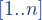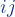# The Max-Cut format

Rules:

• each line starting with a “c” is considered a comment and thus skipped
• the first (non-comment) line contains the key “MAX-CUT” followed by one or more <space> and by two integers n and m [meaning: n  is the number of nodes of the graph, m is the number of edges]
• every following (non-comment) line represents an edge and is described by two integers and a real number i j w, separated by one or more <space>
• i and j are distinct and in the range.  w is the weight of the edge• the number of lines describing the edges of the graph must be equal to m
• loops (i.e., edges with i=j) are simply ignored
• if multiple edges are present, i.e., if the same pair i j appears more than once (irrespective of the order of the two nodes), only one edge is considered with weight equal to the sum of the weights of all such pairs

Example:

Input file:

c
c An instance of MAX-CUT
c
MAX-CUT 4 5
1 2 1
1 3 1.0
1 4 1.0e1
2 3 2
3 4 -1